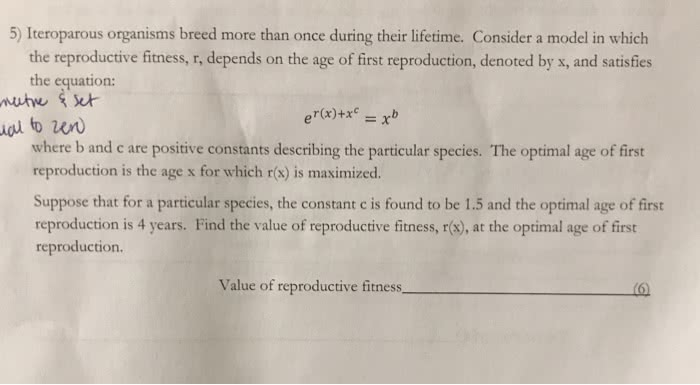1
0
watching
36
views13 Nov 2019

# Need help solving this with step wise process.5oas ganins ic her ictines Cor m i ich the reproductive fitness, r, depends on the age of first reproduction, denoted by x, and satisfies the equation: u to w where b and c are positive constants describing the particular species. The optimal age of first reproduction is the age x for which r(x) is maximized Suppose that for a particular species, the constant c is found to be 1.5 and the optimal age of first reproduction is 4 years. Find the value of reproductive fitness, r(s), at the optimal age of first reproduction. Value of reproductive fitness.